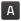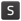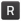In other languages:

Tutorial:Circuit network cookbook

Foreword

This page provides examples of simple circuit network designs and some not so simple designs that others can use, combine and modify. They are designed to be as easy to understand as possible. To see the settings of combinators without opening them, the option "Show combinator settings when detailed info is on" in the graphics options has to be checked and detailed info has to be turned on.

Lamp showing chest content condition

This is the simplest possible use of circuit-network. A lamp is light depending on the number of goods (in this example empty barrels) in a chest.

Setting up circuit connection

• The lamp is connected to the chest
• the lamp is set to light if the chest contain less than 10 empty barrels.

To set the light condition

• Open the lamp (left click on it)
• Set the input to barrels
• Set the operator to < (less than)
• Set the constant number:
• Left click on the constant number
• Move the slider until 10 is shown, or edit the value box directly
• Press set

Depending on the condition you set, the lamp may light if the chest is empty, or if it contains the required quantity of items.

The drawback with this scenario is that the lamp has a white light , and is therefore difficult to differentiate from an ordinary lamp at night.

Oil Setups

Light Oil Cracking

• This circuit provides balanced light oil and petroleum gas production by cracking excess light oil into gas.
• The Pump is connected to the Storage tank by a Red wire.
• The small pump has an enabled condition set to Light Oil > 20000 (nb image is out of date).

Heavy Oil Cracking

• This circuit extends on the previous circuit by adding optional heavy oil cracking to provide lubricant etc.
• The Pump has an enabled condition set to Heavy oil > 20000 (nb image is out of date).

Petroleum split evenly between plastic and sulphuric acid

• This circuit buffers gas in the tank until there is at least 100, then it lets the tank drain until there is less than 50 and the cycle repeats.
• It has a few elements that work together to do achieve this.
• Firstly the Pump is connected to the Wooden chest by a Red wire and the enabled condition on the Pump is set to Raw wood > 0.
• Both of the Inserters are connected to the Storage tank by Red wires.
• The enabled condition on the left inserter is Petroleum gas > 100
• The enabled condition on the right inserter is Petroleum gas < 50.
• You will need to insert a single "Raw wood" into the chest to make it all work.

• It is also possible to do this with decider combinators instead of the inserters, belt and the Wood chest or even just belts.

Lights

Conditional Lights

• In this circuit we connect a series of lamps to a Storage tank.
• By setting different conditions on each lamp we can build an indicator strip.
• The Enabled condition of the first lamp is Petroleum gas > 100.
• The others light up when gas is greater than 200, 300, 400 and 500 respectively.

In this scenario you can connect the storage tank to the lamps directly.

Colored Lights

• To light a lamp with a color rather than white, you need an intermediate device like an Arithmetic combinator that can send a color signal.

Instead of directly connect the the Lamp and the Storage tank you need:
1 Add the Arithmetic combinator.
2 Connect the Storage tank with the input of the Arithmetic combinator.
3 Connect the output of the Arithmetic combinator with the lamp.
4 Set up the Arithmetic combinator:
4.1 Setting the input to Petroleum Gas + 0 (the constant 0 not the signal 0)
4.2 Set the output to the Pink signal (on the bottom row of the last tab of signals.)
5 Set up the lamp:
5.1 Select the "Use colors" check box on the lamp.
5.2 Set the condition to the Pink signal, and what value you want (i.e. > 100)

Misc

Multiple Storages

• If you connect multiple chests to a pole, the pole displays the sum of items in all the chests.
• This also works with Storage tanks and roboports.

Constant combinator

• With a constant combinator you can generate any signals you may need.
• In this example we have generated a signal of 50 Laser turrets and 200 Piercing round magazine.
• Constant combinators are not of much use on their own but we shall use them later.

Logic gates

• In each case the two inputs can be connected with the same color wire or different colors. The inputs are powered by two Constant combinators each of them output an A signal with value 1 for true and nothing or false.
• You can use Decider combinators to make all of the common logic gates.
• The output for each should be set to 1 and the signal of your choice.
• Use the following settings to create different gates:
NOT  A=0
NOR  A=0
NAND A<2
XOR  A=1
AND  A=2
OR   A>0

Constant combinator signs

• You can use Constant combinators to make signs, just set the letter signals in the combinator, each combinator can display 2 characters side by side.

Memory Cell / Counter

• Basic memory cell that counts all the items moved by the inserter
• The Fast inserter is connected to BOTH ends of the arithmetic combinator.
• If the Fast inserter hasn't picked anything up this tick the input to the Arithmetic combinator is the same as and output and hence the values are persisted.
• When the Fast inserter does pick something up its value is added to the output from the previous tick thus incrementing that item.

Multiplier and Dictionaries/Arrays

• Multiplying two signals together is simple and requires only a single combinator, however multiplying a set of signals is more complicated.
• A proof is shown below for the equation and why it works.
• A dictionary is a system that allows a value on a specific signal to be accessed. For example, A can contain many signals (either from a constant combinator or memory cell) and B can contain 1 of a specific signal (such as blue signal). What remains is the blue-signal value from A. This is because all the other signals are multiplied by 0.
• Arrays are similar to dictionaries, but instead of using a signal as a key, we use a number. Constant combinators are placed mapping each signal to a unique number (such as 1 yellow belt, 2 red belt, 3 blue belt, 4 burner inserter, etc). Then, use a combinator of "each = index OUTPUT 1 of each" and plug that in as the input to a dictionary.
((A+B)^2 - (A-B)^2)/4 = AB
(A+B)^2 - (A-B)^2 = 4AB
(A^2 + 2AB + B^2) - (A^2 - 2AB + B^2) = 4AB
4AB = 4AB

Inserters

Limit items placed into a chest

• The Inserter is connected to the Wooden chest using a Red wire.
• The inserter's enabled condition is Advanced Circuit < 10.
• In reality this means the inserter may place more than 10 Advanced circuits in the chest because it could pick up to 3 at once due to stack size bonuses.
• This effect can be even greater with Stack inserters because of their large carrying capacity.
• This technique still gives far greater control than limiting the inventory on the chest.

Balanced chest insert

Goal: Load n chests with approximately the same number of items.

• Place n chests and n inserters.
• Place 1 Arithmetic combinator
• Set the combinator to take Each (yellow star) and divide by the negative number of chests. ie −n.
• Connect all chests to each other and to the input of the combinator using red wire.
• Connect all inserters to each other and to the output of the combinator using red wire.
• Connect each inserter to the box it inserts into with green wire.
• Set the enable condition on each inserter to be Everything (red star) < 0.

The combinator calculates the average number of items in the chests, and makes it negative. Each inserter gets the amount in the chest it is inserting to and adds the negative average, ie it calculates how many more than the average it has in its chest. Thus if that number is negative, it has less than the average in the chest and it enables.

Due to inserter stack bonus the count is not exact. If a precise count is needed, set the inserter stack size to 1.

Balanced Solar panel / Accumulator Production

• This circuit balances production of Solar panels and Accumulators to a desired ratio in my case 24:20.
• The first Arithmetic combinator takes the number of accumulators in the chest and multiplies it by 24.
• The second Arithmetic combinator takes the output of the first combinator and divides it by 20.
• This gives us the number of accumulators that we can directly compare to the number of Solar panels in both inserters.
• If the number of accumulators is greater we enable the Solar panels inserter, if the number of Solar panels is greater we enable the accumulators inserter.
• However, if they are equal, neither machine does anything. So we add a single accumulator to one of the inserters using a constant combinator and a wire of the other color, therefore breaking the deadlock.

Sushi Belts

• Six belts in a row are connected with Red wire and set to Read belts contents and Hold
• This Red wire is then connected to the inserters that insert onto the belt.
• Read hand contents is unselected for all inserters.
• Mode of operation is set to Enable/Disable on all inserters.
• The first inserter is enabled when Science pack 1 = 0
• The other inserters are set similarly for the other science packs.

Memory Cell Design

• This circuit counts the number of items of each type on a looping belt by counting the numbers that are added and removed from the belt by inserters.
• Each inserter that takes items off the belt is connected together with Red wire and each of these inserters is set to Mode of operation none, Read hand content selected and Hand read mode pulse.
• These inserters are connected to the input of the left arithmetic combinator.
• The left Arithmetic combinator multiples each input by -1 and outputs it to each.
• The right Arithmetic combinator is a memory cell as above.
• The memory cell's input is connected to the inserters that are placing items on the belt and the output of the left Arithmetic combinator.
• The inserters that place items onto the belt have an enabled condition that is based on the number of items on the belt.

Power

Optimal usage of fuel for nuclear power

Unlike the normal steam power that adjusts fuel usage based on power usage, the nuclear reactors spend fuel in fixed units of time. To be exact, the consumption of 1 fuel cell takes exactly 200 seconds.

Combined with the fact that creating the nuclear fuel cells are time consuming and expensive to create, it is therefore beneficial to optimize their use to match the actual consumed power.

The above picture shows a setup with 4 reactors, that spend only 1 fuel cell each whenever steam runs low.

There are a few elements in this setup:

• Storage tank that provides the Steam signal. You should only read from one storage tank, and it should have pipe connections to all your other steam storage tanks.
• Chests containing Uranium fuel cells for the reactor.
• Output inserters that take Empty fuel cells from the reactor. This is connected to the storage tank to listen for the steam signal, and to the chests to listen for the uranium fuel cell signal. If the steam level is low and there are uranium fuel cells available, it removes the empty fuel cells from the reactor and sends an empty fuel cell signal (since "Read hand contents" is checked).
• Input inserters that put uranium fuel cells into the reactor. This is connected to the output inserters and listens for the empty fuel cell signal. The "Override stack size" is set to 1, so that it only inserts 1 fuel cell at a time.

Since this design uses empty fuel cells as a signal to fill the reactor, you need to manually insert 1 uranium fuel cell into the reactor to get it started.

Latches

RS latch - single decider version

This discussion on the Factorio forums starts with the common 2 decider RS latch version, but the thread goes on to explain why this single decider version is better. In the thread, the latch is described as an SR latch. However, when both inputs are true, the latch will reset, so it is an RS latch.

Backup steam example

This example will turn on the steam generator when the Accumulator charge drops to 20%, but will "latch" (remember) the On state until the accumulator is charged to 90%.

Latching is used to introduce hysteresis and avoid the power switch rapidly cycling on and off (as the accumulator falls to 19%, charges to 20%, falls to 19% and so on).Copy blueprint string

Accumulator outputs the current charge level as % on signalFirst decider outputs "Set" (= 1) if Accumulator is less than 20%.

Second decider outputs "Reset" (= 1) once Accumulator is more than 90% full.

RS Latch configuration

The central decider and green feedback wire is the actual RS Latch. It latches the Set signaluntil the Reset signalis received (and vice-versa).
NB: the latch expects binary inputs (&must be 0 or 1) - this is why the previous two deciders are required.
When both inputs are true, the reset signal takes priority and the latch resets. This means it is an RS latch instead of an SR latch.

The Power switch isolates the generator from the rest of the factory until= 1

RS latch

• This should be familiar to anyone with any background in electronics.
• The signal is set and reset with the constant combinators on the left by setting an A=1 signal.
• The latch "remembers" which one was last set and the light stays on until another signal is received.

Usage of RS latch

• Here is an example of how you could use an RS latch.
• The two extra Decider combinators provide the set and reset conditions.
• Petroleum gas < 50 and petroleum gas > 100.

Belt only latch

• To make it work, 3 pieces of raw wood must be placed on the inside lane of the belt.
• It will have higher latency than the combinator version, but in most situations you will not notice the difference.

Displays

Numerical Display

• Each digit is driven by its own Green wire, that wire holds 15 signals, one for each lamp used in the digit.
• Constant combinators are used to define which lamp should light up for each value.

Black and White Grid Display

• Each row has its own Red wire connection and within that row each light has a numbered signal 0-9.
• We turn each light on by just setting or clearing the relevant signal.

Multicolor Display by DaveMcW

• To understand how this works, you first need to understand how color lights choose which color to light up when there are multiple colored signals.
• The lamp will light up with the colored signal that is greater than zero and earliest in this list: Red, Green, Blue, Yellow, Pink, Cyan, White.
• We have a Red wire per column, that wire has each of the colored signals on it at different values and a numbered signal for each row.
• There is a Arithmetic combinator for each cell that subtracts the "row" value from each of the colored signals.
• And this enables us to choose the color for each cell.
• Simple!